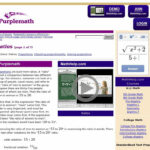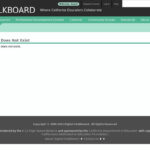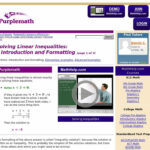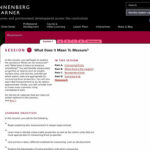# Tag Archives: mathematics#### Do you Measure Up? Area and Perimeter

September 14th, 2017

This lesson is the first in a series of four lessons which will help build an understanding of area and perimeter by using real world applications in order to build a skateboard park.#### Creating an Immigrant Scorecard – Creating a Formula

September 14th, 2017

In this lesson, students will consider and propose various factors that can be used to make decisions regarding prospective immigrants to the United States of America. Students will conduct public opinion research to determine which factors should be used, and the relative weighting that each of these factors should receive, in creating an Immigrant Scorecard.#### From Ellis Island to the Trump Wall – A Mathematical Analysis

September 14th, 2017

Juxtaposing the architecture of Ellis island with that of the proposed Trump Wall creates many opportunities for mathematical analysis and frames a discussion of how our nation’s attitude toward immigration has changed#### Ratios

September 14th, 2017

This set of instructional materials and problems helps students understand ratios and proportions, starting with a simple review and progressing into the more advanced territory of similar triangles#### A Geometric Proof of the Pythagorean Theorem

September 14th, 2017

Students will see an animated presentation of the proof of the Pythagorean Theorem. This animated PowerPoint presentation uses shearing and the invariance of the area of triangles with congruent bases and heights to show a step-by-step geometric proof of the Pythagorean Theorem.#### Trig River

September 14th, 2017

Students learn about and use a right triangle to determine the width of a “pretend” river. Working in teams, they estimate of the width of the river, measure it and compare their results with classmates.#### Linear Functions

September 14th, 2017

In this tutorial, “Linear functions of the form f(x) = a x + b and the properties of their graphs are explored interactively using an applet.” The applet allows students to manipulate variables to discover the changes in intercepts and slope of the graphed line. There are six questions for students to answer, exploring the […]#### Pizza Fractions

September 14th, 2017

The classroom poster helps students understand fractions as parts of a whole by illustrating pizzas and fractions of pizzas. These eight images show a whole pizza, a pizza cut in half and in quarters with various pieces removed. It is an excellent visual to present students as they dive into more complex understanding of fractions […]#### Solving Linear Inequalities: Introduction and Formatting

September 14th, 2017

From Elizabeth Stapel at Purplemath, this module helps students understand how to solve linear inequalities with an introduction to the topic using both inequality notation and a graphic representation to express the inequality.#### What Does It Mean To Measure?

September 14th, 2017

In this session, students will begin to explore the questions “What can be measured?” and “What does it mean to measure something?” Students identify measurable properties of objects such as weight, surface area, and volume, and discuss which metric units are appropriate for measuring these properties.